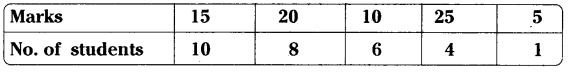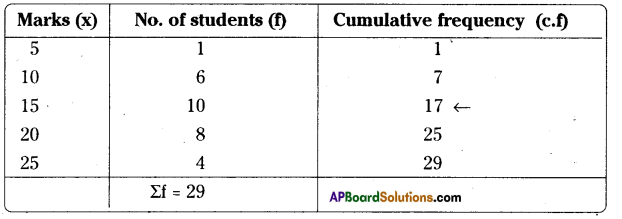AP State Syllabus AP Board 9th Class Maths Solutions Chapter 9 Statistics InText Questions and Answers.

## AP State Syllabus 9th Class Maths Solutions 9th Lesson Statistics InText QuestionsActivity

Divide the students of your class into four groups. Allot each group the work of collecting one of the following kinds of data: [Page No. 195]
Solution:
i) Weights of all the students in your class
ii) Number of siblings that each student have.
iii) Day wise number of absentees in your class during last month.
iv) The distance between the school and home of every student of your class.Do This

Which of the following are primary and secondary data ? (Page No. 195)
i) Collection of the data about enroll¬ment of students in your school for a period from 2001 to 2010.
Solution:
Secondary data.

ii) Height of students in your class re¬corded by physical education teacher.
Solution:
Primary data.Activity

Make frequency distribution table of the initial letters of that denotes surnames of your classmates and answer the following questions.
i) Which initial letter occured mostly among your classmates?
iii) Which letter occured least as an initial among your classmates? [Page No. 197]

Think, Discuss anil Write

Question 1.
Give 3 situations, where mean, median and mode are separately appropriate and counted. [Page No. 202]
Solution:
Mean :
a) Rice required for a certain number of students for a given period.
b) To compare the marks of students of a class.
c) To calculate the daily income of a shop during a month.

Median :
a) To study the salaries of staff of an institution.
b) To compare heights of boys and girls.

Mode :
a) To find the size of the shoe with heighest sale.Try These

Question 1.
Find the median of the scores 75, 21, 56, 36, 81, 05, 42. [Page No. 207]
Solution:
Arranging the data in ascending order
05, 21, 36, 42, 56, 75, 81
Number of terms in the data = 7 – odd
∴ $$\left(\frac{n+1}{2}\right)^{t h}$$ term = $$\frac{7+1}{2}=\frac{8}{2}$$ = 4th term is the median = 42.

Question 2.
Median of a data arranged in ascending order 7, 10, 15, x, y, 27, 30 is 17 and when one more observation 50 is added to the data the median has become 18. Find x and y. [Page No. 207]
Solution:
The given data in ascending order is 7, 10, 15, x, y, 27, 30
Median = $$\left(\frac{n+1}{2}\right)^{t h}$$ term = $$\left(\frac{7+1}{2}\right)$$ = 4th term = x
∴ x = 17 by problem
When 50 is added, the data becomes 7, 10, 15, 17, y, 27, 30, 50
Median = $$\frac{\left(\left(\frac{n}{2}\right)+\left(\frac{n}{2}+1\right)\right)}{2}$$ terms
18 = $$\frac{17+y}{2}$$ (by problem)
17 + y = 36
y = 36 – 17 = 19Question 3.
Find the median marks in the data.[Page No. 208]Solution:Median = $$\left(\frac{n+1}{2}\right)^{t h}$$ term as N = 29 is odd
∴ $$\frac{29+1}{2}$$ = 15th term.
15th observation is 15. (from the table)

Question 4.
In finding the median, the given data must be in order ? Why ? [Page No. 208]
Solution:
In finding the median, the observations must be in order. The data is to be arranged either in ascending/descending order to divide the data into two equal groups.Think and Discuss

Question 1.
Classify your classmates according to their heights and find the mode of it.
(Page No. 208)
Solution:
Student to find the mode of the classmates according their heights.

Question 2.
If shopkeeper has to place an order for shoes, which number shoes should he order more ? [Page No. 208]
Solution:
Number 7 as it has highest sales.